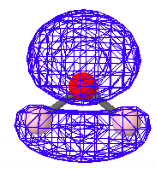Skip to main content

# 164: Two Perspectives on the Bonding in Water

Chemists use a variety of models to describe the electronic structure of molecules. The oldest and most rudimentary model which is still in widespread use, and is taught at all levels of the chemistry curriculum, is the localized electron pair model proposed by G. N. Lewis in 1916. This pre-quantum approach to chemical bonding was stimulated in part by the observation that half the elements in the periodic table have an odd number of electrons, but very few molecules do.

Today we know that the importance of the electron pair is explained by the Pauli Exclusion Principle. Electrons, as fermions, cannot have the same set of quantum numbers. Therefore, if the three spatial quantum numbers are the same the spin quantum number must be different, and the spin quantum number has only two allowed values - plus or minus 1/2. Thus only two electrons can occupy an orbital - a region of space.

Molecular orbital theory, a successor to the Lewis model, is based on the Exclusion Principle and the postulates of quantum mechanics. Thus it retains the concept of the electron pair but, due to the wave nature of quantum theory, it abandons spatial localization. This is perhaps the most challenging concept that quantum mechanics offers chemists, the delocalization of the electron pair over the entire molecule.

To emphasize the difference between the Lewis and MO approaches the bonding in water will be considered. The traditional Lewis structure for water is shown below. There are two equivalent bonding electron pairs and two equivalent non-bonding electron pairs.Therfore, according to the Lewis localized electron pair bonding model there should be two bands in the experimental photoelectron spectrum of equal weight. However, the actual spectrum shows four distinct bands, indicating that there are four types of valence electrons in the water molecule, not two.

The quantum mechanical treatment of bonding is based primarily on the LCAO-MO approach where a molecular orbital is formed as a linear combination of atomic orbitals. A quantum mechanical calculation for water yields the following valence electron molecular orbitals. Note that there are four distinctly different molecular orbitals in agreement with the spectroscopic data mentioned above. Note also that the electron density is distributed over the molecule as a whole.

The canonical molecular orbitals are arranged on a relative energy scale below with comments.Highest Occupied Molecular Orbital - HOMO: Non-bondingHOMO-1: Also non-bonding with regard to oxygen and hydrogenHOMO-2: Bonding - charge density present between oxygen and hydrogen.HOMO-3: Bonding-charge density present between oxygen and hydrogen.

The fact that the delocalized molecular orbitals are in agreement with experimental data but the localized electron pair model is not presents a challenge for the chemist who is accustomed to placing confidence in the value of the Lewis model. However, the quantum mechanical superposition principle allows one to construct localized molecular orbitals (LMOs) from the canonical delocalized molecular orbitals (DMOs). In other words, quantum mechanics itself provides the justification for placing confidence in the utility of the Lewis electron-pair model.

There are a variety of computational protocols for generating LMOs from DMOs. The following LMOs were calculated using a method developed by Ruedenberg in which intra-orbital electron repulsions are maximized. It is clear that the four LMOs generated by this protocol bear a strong relationship to the simple localized electron pair model of Lewis for water shown above.

Bonding LMOsNon-Bonding LMOs• Was this article helpful?